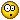News: Check out the originating "3d Mandelbulb" thread here## The All New FractalForums is now in Public Beta Testing! Visit FractalForums.org and check it out!

 Pages:    Go DownAuthor Topic: Area of Julia Set  (Read 1577 times) Description: research 0 Members and 1 Guest are viewing this topic.
hgjf2
Fractal PhenomPosts: 456« on: June 20, 2013, 10:02:56 AM »

Yet , neither scientist could find a formula for area of JULIA SET , just by formula {z|P(P(P...P(z)...))<>infinity, P(z) = z*z+c}.Logged
hgjf2
Fractal PhenomPosts: 456« Reply #1 on: June 20, 2013, 10:09:04 AM »

Yet , neither scientist could find a formula for area of JULIA SET , just by formula {z|P(P(P...P(z)...))<>infinity, P(z) = z*z+c}.But I surprised that for c=-1 from formula {z|P(P(P...P(z)...))<>infinity, P(z) = z*z+c}, area of this Julia Set seem that give 1,4142135623... , and I know is equal with sqrt2.I knew after I used VISUAL BASIC and C# for calculate this area with MONTE CARLO METHOD and filling space and comparing method because I don't know neither math formula for JULIA SET quadrating and calculate area. But I don't stand how the area have given sqrt2 for P(z) = z*z-1.Logged
Pauldelbrot
Fractal SeniorPosts: 2592« Reply #2 on: June 20, 2013, 11:06:09 AM »

This colors the Mandelbrot set interior by the areas of Julia sets. The UF gradient is mapped to the range of areas from 0 to pi (the area of the Julia set at (0,0), which seems to be close to the maximum attained).

Code:
JuliaArea {
final:
int ct = 0
int p = 0
int seed = 385472
WHILE (p < @julpoints)
int i = 0
seed = random(seed)
float r = 2*(seed/#randomrange)
seed = random(seed)
complex x = r + (0,2)*(seed/#randomrange)
WHILE (i < @juliters && |x| < 2)
x = sqr(x)+#pixel
i = i + 1
ENDWHILE
IF (|x| < 2)
ct = ct + 1
ENDIF
p = p + 1
ENDWHILE
#index = (ct*16)/(#pi*@julpoints)
default:
title = "Julia Area"
param julpoints
caption = "Number of samples"
default = 3000
min = 1
endparam
param juliters
caption = "Julia maximum iterations"
default = 30
min = 1
endparam
}

 « Last Edit: June 20, 2013, 11:07:40 AM by Pauldelbrot »Logged

matsoljare
Fractal LoverPosts: 215« Reply #3 on: June 20, 2013, 10:59:32 PM »

Yeah, that is pretty much the same as the "Supermandelbrot" rendering that i proposed a couple of years ago, not much have been done of it though:

http://www.fractalforums.com/new-theories-and-research/supermandelbrot-and-superjulia-imagingLogged
hgjf2
Fractal PhenomPosts: 456« Reply #4 on: June 21, 2013, 10:43:20 AM »

Nice rendering on MANDELBROT SET graphic where the color show value of area of Julia set at each point.
If making same algorithm on cubic Julia Set  {z|P(P(P...P(z)...))<>infinity;P(z) = z*z*z+z*z+c}, the graphic cover two cubic Mandelbrot sets have at base formula fc(z)=z*z*z+z*z+c with starting from z=0 and z=-22/27 whick is simetrical , one is another mirrored by the ax {z=a-(1/3)i;\/a<-R}

LEGEND OF MATH SYMBOLS: \/  anything to be ;    <- apartening ;  <> different; R  set of real numbersLogged
Pauldelbrot
Fractal SeniorPosts: 2592« Reply #5 on: June 21, 2013, 12:07:58 PM »

Nice rendering on MANDELBROT SET graphic where the color show value of area of Julia set at each point.

Thanks.Logged

 Pages:    Go Down
 Related Topics Subject Started by Replies Views Last postZoom into flat area, looking across Mandelbulb Renderings BradC 3 1355December 17, 2009, 01:55:36 PM by kram1032Two Entries In Wrong Area 3rd Annual FractaSpring 2010 Fractal Art Competition (Completed) « 1 2 » Nahee_Enterprises 17 4486May 03, 2010, 05:58:22 PM by Bent-Winged AngelIncubation area Mandelbulb3D Gallery bib 0 478March 04, 2012, 12:09:41 AM by bibAre there any Mandelbulb veterans in the Los Angeles area? Mandelbulb 3d Jhaptal 2 806August 06, 2013, 11:45:02 PM by JhaptalArea of fractals - Area of quasifuchsian grandma fractals (new) Theories & Research hgjf2 7 663July 26, 2014, 02:14:22 PM by hgjf2